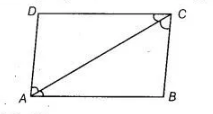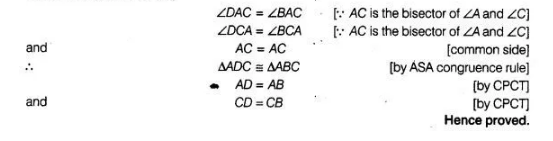# If ABCD is a quadrilateral such that diagonal`
Question:

If ABCD is a quadrilateral such that diagonal AC bisects the angles A and C,then prove that AB = AD and CB = CD.

Solution:

Given in a quadrilateral $A B C D$, diagonal $A C$ bisects the angles $A$ and $C$.To prove $A B=A D$ and $C B=C D$

Proof in $\triangle A D C$ and $\triangle A B C$,